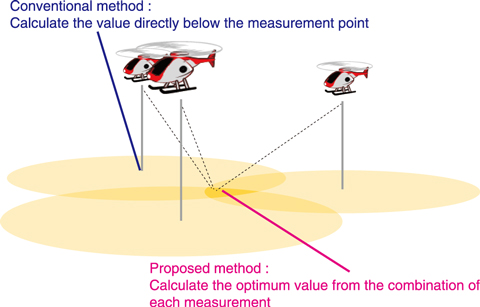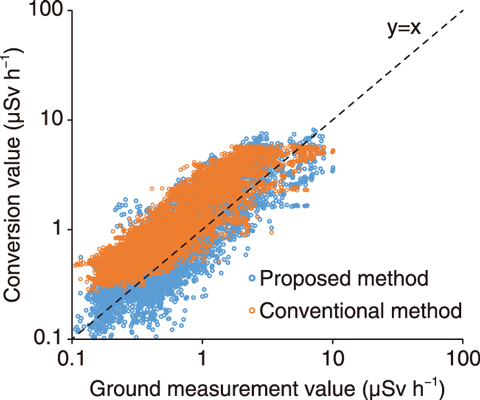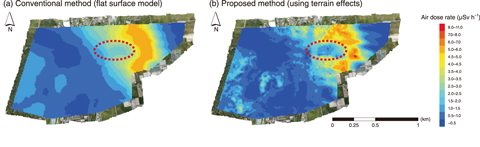# 1-15 Quickly and Accurately Measuring Environmental Radiation LevelsFig.1-35 Conventional and proposed radiation measurement method

The conventional method calculates ground radiation values directly below the measurement point (i.e., point-to-point). The proposed method calculates ground radiation using multiple measured and terrain data (i.e., multi-point-to-point).Fig.1-36 Comparison between conversion value measurement from the sky and ground measurement value

The actual and calculated dose rate 1 m above the ground using the conventional and proposed methods are shown.This Picture(175kB)

Fig.1-37 Contour maps of the calculated air dose rate

Obtained via the (a) conventional and (b) proposed methods. Both convert to 1 m above ground air dose rate.

Since the accident at the TEPCO’s Fukushima Daiichi NPS (1F), manned and unmanned helicopters have been used to monitor the radiation levels around the 1F, which are then converted to indicate the dose rate at 1 m above the ground. The conventional conversion method assumes a flat topography and uniform distribution of the radiation, and uses an attenuation factor of radiation by air at the distance between the measurement point and the ground. Therefore, conversion result of actual place, which differs from this assumption, does not match with real ground measurement data. This is especially true in areas of uneven geographical features, shielding by a tree or building, and patchy radiation distribution. An analysis method using the inverse analysis method was thus developed to consider the effects of geographical features and forest shielding in the calculation of dose rate.

The maximum likelihood-expectation maximization (ML-EM) method, used to visualize internal organs in the medical radiation field, was applied. In this method, the optimal distribution of ground values is calculated from many radiation values measured from the sky (Fig.1-35). An attenuation factor between the radiation source and the detector is calculated by taking into account the thickness of air, trees, and soil based on actual photogrammetry data.

The resulting radiation levels obtained with an unmanned helicopter using the conventional and proposed method are shown in Fig.1-36. Conversion using the proposed inverse analysis method obtained more accurate values than did the conventional method, thus improving the accuracy of conversion from the sky to the ground. Contour maps of the air dose rate obtained by the (a) conventional and (b) inverse analysis method are shown in Fig.1-37, where the red circled area shows a decontaminated area. In the conventional method, the decontaminated area was not clearly visible due to the influence of surrounding radiation, whereas the proposed method more clearly shows the decontamination.

Future work will aim to optimize the parameters of the proposed method and develop an algorithm allowing it to be applied on measurements from both the sky and ground.

| | | | |